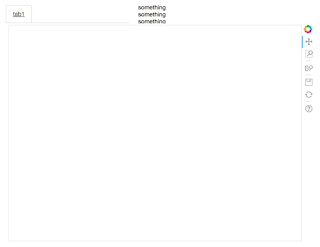# Tab is smaller than the figure it contains when using Layout

I have the following code:

``````from bokeh.plotting import figure
from bokeh.models import Div
from bokeh.layouts import layout
from bokeh.models.widgets import Panel, Tabs
from bokeh.plotting import curdoc

fig = figure(plot_width=700, plot_height=500)

div = Div(width=1000)

div.text = "something<br>something<br>something<br>something"

tab1 = Panel(child=row(fig), title="tab1")

tabs = Tabs(tabs=[ tab1 ])

layout([[tabs,div]])
)

``````

``

The output looks like this (Python 3.4, Bokeh 0.12.5, Mac OS 10.11.6, running in server mode in Chrome):Somehow, tabs cannot contain the figure so that the figure and div clash with each other in layout.

Did I do something wrong? Or is this a bug?